Homework Help Question & Answers

# QUESTION 17 Which of the following transitions (in a hydrogen atom) represent emission of the longest...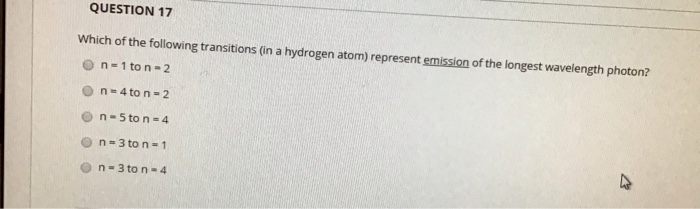QUESTION 17

Which of the following transitions (in a hydrogen atom) represent emission of the longest wavelength photon?

$$n=1$$ to $$n=2$$

$$n=4$$ to $$n=2$$

$$n=5$$ to $$n=4$$

$$n=3$$ to $$n=1$$

$$n=3$$ to $$n=4$$

#### Homework Answers

Answer #2 ✔ Recommended Answer

n = 5 to n=4

answered by: cry
Add a comment
Answer #1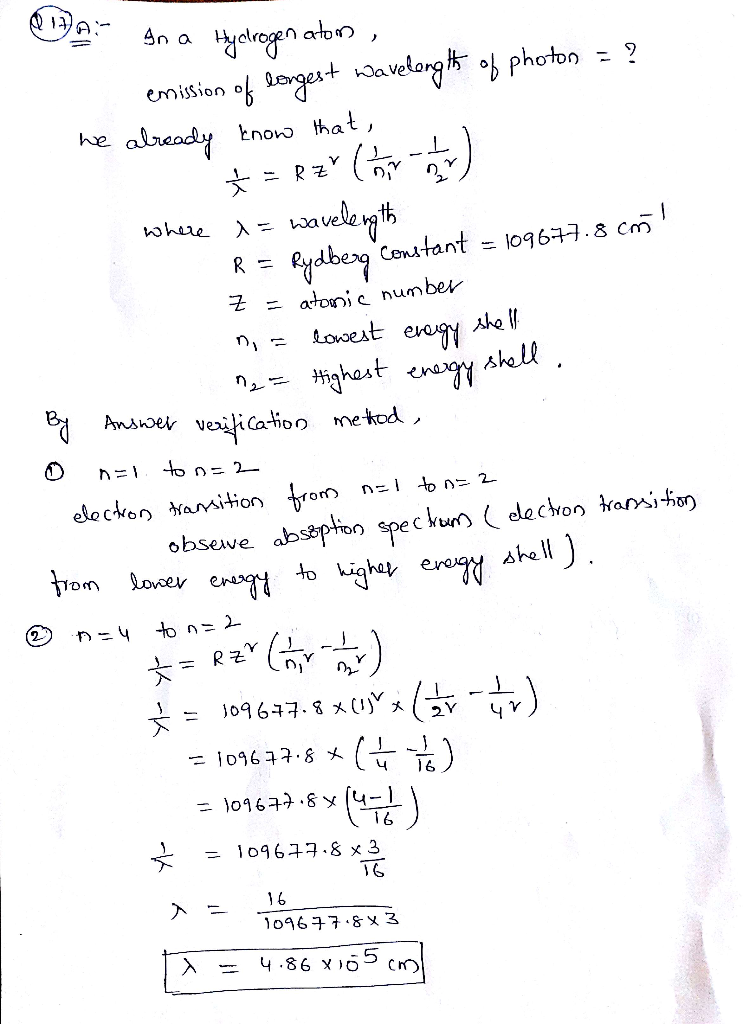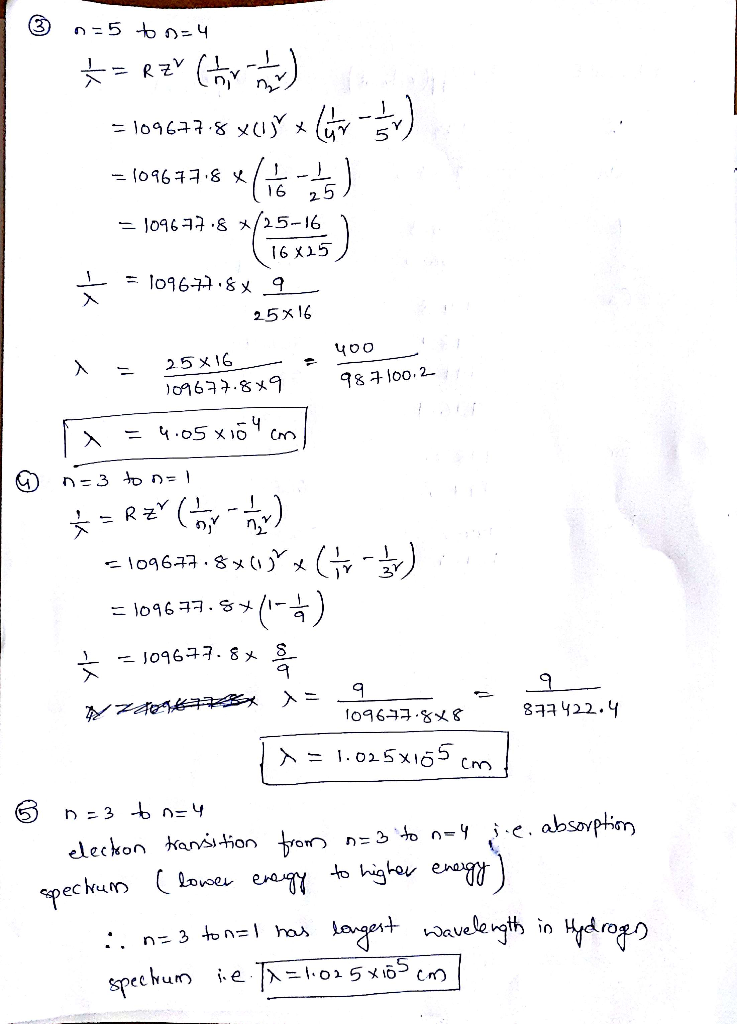Know the answer?
Your Answer:

#### Post as a guest

Your Name:

What's your source?

#### Earn Coin

Coins can be redeemed for fabulous gifts.

Not the answer you're looking for? Ask your own homework help question. Our experts will answer your question WITHIN MINUTES for Free.
Similar Homework Help Questions
• ### Of the following transitions in the hydrogen atom, the __________ transition results in the emission of...

Of the following transitions in the hydrogen atom, the __________ transition results in the emission of the photon with the shortest wavelength. A) n = 1 → n = 5 B) n = 5 → n = 1 C) n = 5 → n = 2 D) n = 3 → n = 5 E) n = 1 → n =4

• ### Question 41 (1 point) For which of the following electron transitions would a hydrogen atom emit...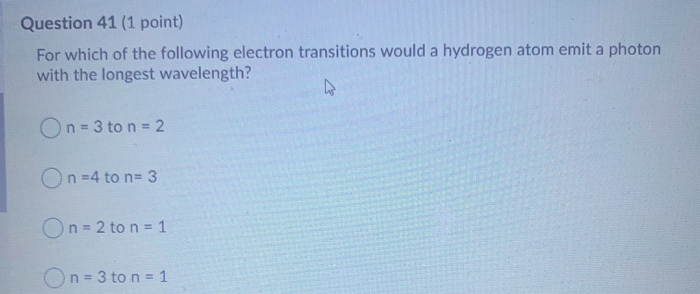Question 41 (1 point) For which of the following electron transitions would a hydrogen atom emit a photon with the longest wavelength? On = 3 to n = 2 On=4 to n=3 On = 2 to n = 1 On = 3 to n = 1

• ### transition Of the following transitions in the Bohr hydrogen atom, the_ results in the emission of...transition Of the following transitions in the Bohr hydrogen atom, the_ results in the emission of the lowest-energy photon. When the electron in a hydrogen atom moves from n = 6 ton = 2, light with a wavelength of nm is emitted.

• ### Determine which of the following H atom electron transitions has the longest wavelength and which has the shortest...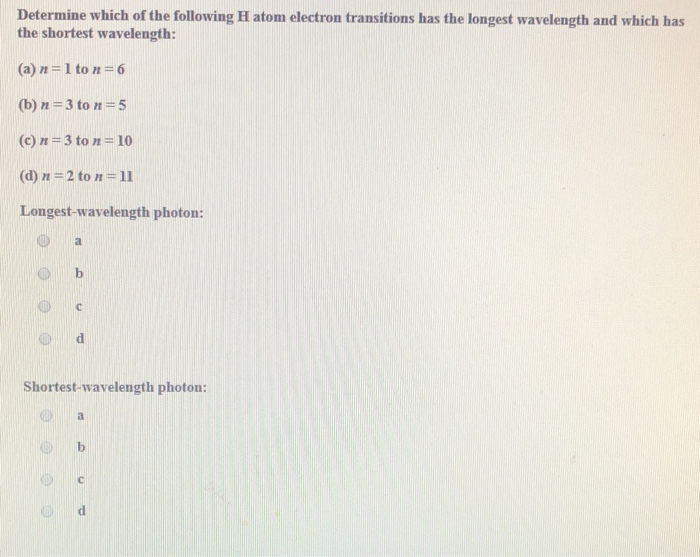Determine which of the following H atom electron transitions has the longest wavelength and which has the shortest wavelength: (a) n=1 to n=6 (b) n=3 to n=5 (c) n=3 to n=10 (d) n = 2 to n=11 Longest-wavelength photon: Od Shortest-wavelength photon:

• ### The following is a diagram of energy states and transitions in the hydrogen atom -n infinity...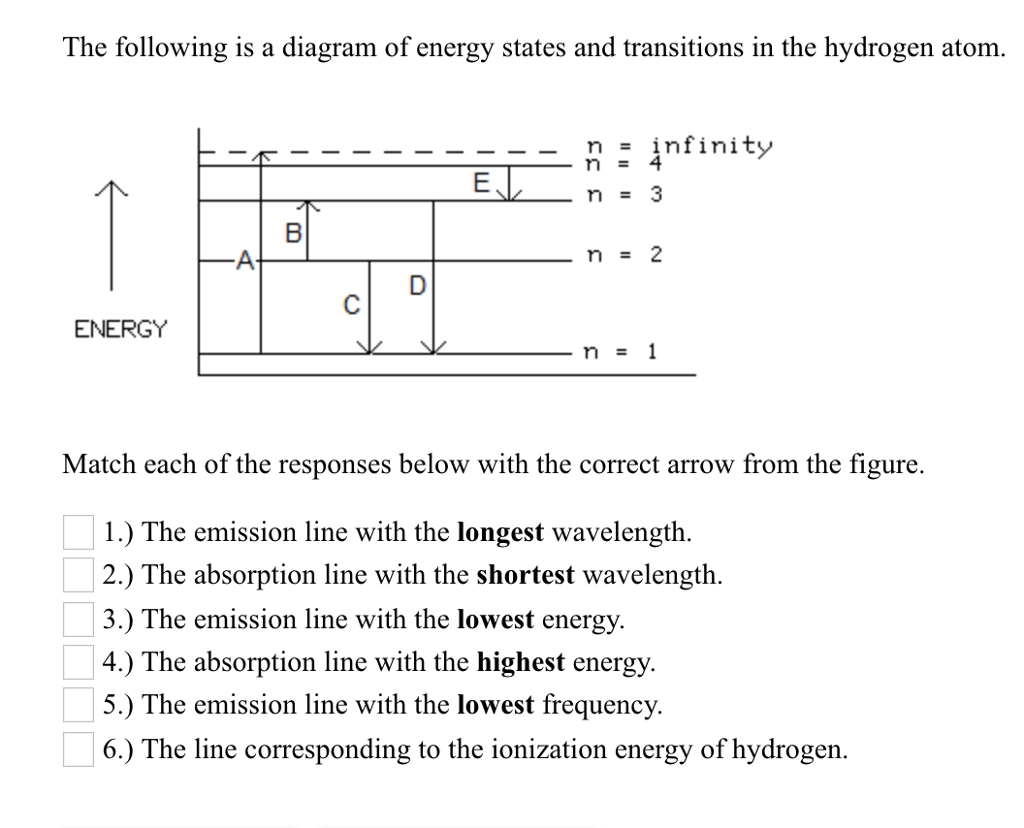The following is a diagram of energy states and transitions in the hydrogen atom -n infinity ENERGY Match each of the responses below with the correct arrow from the figure. 1.) The emission line with the longest wavelength. 2.) The absorption line with the shortest wavelength. 3.) The emission line with the lowest energy 4.) The absorption line with the highest energy 5.) The emission line with the lowest frequency. 6.) The line corresponding to the ionization energy of hydrogen.

• ### The following is a diagram of energy states and transitions in the hydrogen atom. no infinity...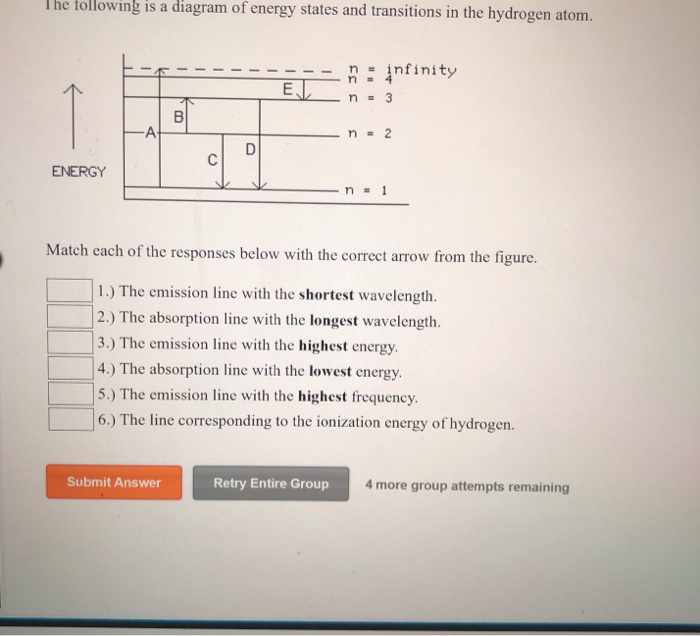The following is a diagram of energy states and transitions in the hydrogen atom. no infinity 33 33 n = 3 = 2 ENERGY n = 1 Match each of the responses below with the correct arrow from the figure. 1.) The emission line with the shortest wavelength. 2.) The absorption line with the longest wavelength. 3.) The emission line with the highest energy. 4.) The absorption line with the lowest energy. 5.) The emission line with the highest frequency....

• ### O c 646 nm o d. 103 nm O e 155 nmm QUESTION 7 Which of...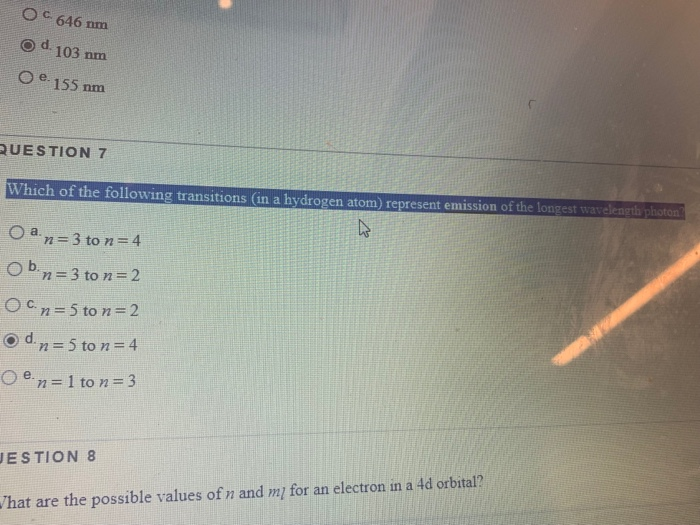O c 646 nm o d. 103 nm O e 155 nmm QUESTION 7 Which of the following transitions (in a hydrogen atom) represent emission of the longest wavelength photon n 5 to n- 2 d. ESTION 8 hat are the possible values of n and mi for an electron in a 4d orbital?

• ### 7. Of the ultraviolet transitions in the Bohr hydrogen atom, which transition results in the emission...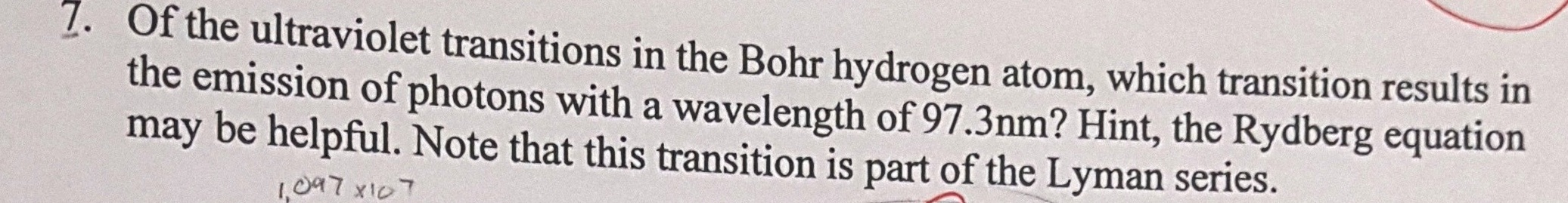7. Of the ultraviolet transitions in the Bohr hydrogen atom, which transition results in the emission of photons with a wavelength of 97.3nm? Hint, the Rydberg equation may be helpful. Note that this transition is part of the Lyman series. 1,097x107

• ### 4. Which of the following transitions of an electron in a hydrogen atom corresponds to the...

4. Which of the following transitions of an electron in a hydrogen atom corresponds to the lowest frequency of the emitted photon? (n = 2 → n = 10 means a transition from the state with n = 2 to the state with n = 10) (A) n = 4 → n = 2 (B) n = 5 → n = 3 (C) n = 4 → n = 5 (D) n = 6 → n = 3 (E) n...

• ### HW ASTR 152

Considering the transitions in a hydrogen atom, which transition results in the emission of a photon with the longest wavelength?n=4   ---->   n=1n=1   ---->   n=4n=5   ---->   n=1n=2   ---->   n=5n=3   ---->   n=5Next